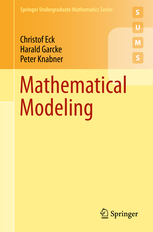Mathematical Modeling

Christof Eck, Harald Garcke, Peter Knabner:

Mathematical Modeling

Springer Undergraduate Mathematics Series. Springer International, Cham 2017, ISBN: 978-3-319-55160-9.The flyer can be found here.

Summary:

Mathematical models are the decisive tool to explain and predict phenomena in the natural and engineering sciences. With this book readers will learn to derive mathematical models which help to understand real world phenomena. At the same time a wealth of important examples for the abstract concepts treated in the curriculum of mathematics degrees are given. An essential feature of this book is that mathematical structures are used as an ordering principle and not the fields of application.
Methods from linear algebra, analysis and the theory of ordinary and partial differential equations are thoroughly introduced and applied in the modeling process. Examples of applications in the fields electrical networks, chemical reaction dynamics, population dynamics, fluid dynamics, elasticity theory and crystal growth are treated comprehensively.

1. Introduction
1.1 What Do We Mean by (Mathematical) Modeling?
1.2 Aspects of Mathematical Modeling: Example of Population Dynamics
1.3 Population Models with Restricted Resources
1.4 Dimensional Analysis and Scaling
1.5 Asymptotic Expansions
1.6 Applications from Fluid Mechanics
1.7 Literature
1.8 Exercises
2. Systems of Linear Equations
2.1 Electrical Networks
2.2 Space Frames
2.3 Constrained Optimization
2.4 Literature
2.5 Exercises.
3. Basic Principles of Thermodynamics
3.1 The Model of an Ideal Gas and the Maxwell–Boltzmann Distribution
3.2 Thermodynamic Systems and the Thermodynamic Equilibrium
3.3 The First Law of Thermodynamics
3.4 The Second Law of Thermodynamics and the Notion of Entropy
3.5 Thermodynamic Potentials
3.6 The Legendre Transform
3.7 The Calculus of Differential Forms
3.8 Thermodynamics of Mixtures and the Chemical Potential
3.9 Chemical Reactions in Multi Species Systems
3.10 Equilibria of Chemical Reactions and the Mass Action Law
3.11 Kinetic Reactions
3.12 Literature
3.13 Exercises
4. Ordinary Differential Equations
4.1 One-Dimensional Oscillations
4.1.1 Forced Oscillations
4.2 The Lagrangian and Hamiltonian Form of Mechanics
4.3 Examples from Population Dynamics
4.4 Qualitative Analysis, Phase Portraits
4.5 The Principle of Linearized Stability
4.6 Stability of Linear Systems
4.7 Variational Problems for Functions of One Variable
4.8 Optimal Control with Ordinary Differential Equations
4.9 Literature
4.10 Exercises
5. Continuum Mechanics
5.1 Introduction .
5.2 Classical Point Mechanics
5.3 From Particle Mechanics to a Continuous Medium
5.4 Kinematics
5.5 Conservation Laws
5.6 Constitutive Relations
5.7 The Second Law of Thermodynamics in Continuum Mechanics
5.8 Principle of Frame Indifference
5.9 Constitutive Theory for Viscous Fluids
5.10 Modeling of Elastic Solids
5.11 Electromagnetism
5.12 Dispersion
5.13 Literature
5.14 Exercises
6. Partial Differential Equations
6.1 Elliptic Equations
6.1.1 Calculus of Variations
6.1.2 The Fundamental Solution
6.1.3 Mean Value Theorem and Maximum Principles
6.1.4 Plane Potential Flows, the Method of Complex Variables
6.1.5 The Stokes Equations
6.1.6 Homogenization
6.1.7 Optimal Control of Elliptic Differential Equations
6.1.8 Parameter Identification and Inverse Problems
6.1.9 Linear Elasticity Theory
6.2 Parabolic Equations
6.2.1 Uniqueness of Solutions, the Energy Method
6.2.2 Large Time Behavior
6.2.3 Separation of Variables and Eigenfunctions
6.2.4 The Maximum Principle
6.2.5 The Fundamental Solution
6.2.6 Diffusion Times
6.2.7 Invariant Transformations
6.2.8 General Initial Data
6.2.9 Brownian Motion
6.2.10 Traveling Waves
6.2.11 Reaction Diffusion Equations and Traveling Waves
6.2.12 Turing Instability and Pattern Formation
6.2.13 Cahn–Hilliard Equation and Pattern Formation Processes
6.3 Hyperbolic Conservation Laws
6.4 The Wave Equation
6.5 The Navier–Stokes Equations
6.6 Boundary Layers
6.7 Literature
6.8 Exercises
7.  Free Boundary Problems
7.1 Obstacle Problems and Contact Problems
7.2 Free Boundaries in Porous Media
7.3 The Stefan Problem
7.4 Entropy Inequality for the Stefan Problem
7.5 Undercooled Liquids
7.6 Gibbs–Thomson Effect
7.7 Mullins–Sekerka Instability
7.8 A Priori Estimates for the Stefan Problem with Gibbs–Thomson Condition
7.9 Phase Field Equations
7.10 Free Surfaces in Fluid Mechanics
7.11 Thin Films and Lubrication Approximation
7.12 Literature
7.13 Exercises

Appendix A: Function Spaces
Appendix B: Curvature of Hypersurfaces
References
Index